Q:

# Longest Common Subsequence

Given two strings str1 and str2, find length of the longest common sub-sequence between them

```    Let the strings be
str1="includehelp"
str2="letsinclude"

Output will be:
Longest common sub-sequence length is 7
The longest common sub-sequence is: "include"
```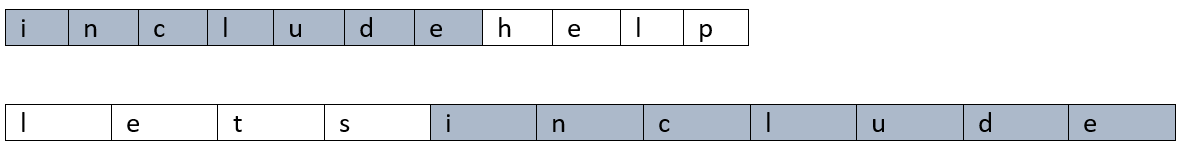The output is given above where the longest common sub-sequences is in same colour.

The problem can be solved in a brute-force way. By generating all sub-sequences and checking them whether equal or not. Finally taking the longest common subsequence. But undoubtedly this is not at all computable since generating all sub-sequence is itself exponential and then permutations for checking any two sub-sequences.

The recursive way to solve is

Let,

```        l1 = Length of the first string,str1
l2 = Length of the second string,str2

f(l1,l2) = Longest common subsequence length for string lengths l1 & l2
```

Now,

Think of the following example,

Say first string is: x1 x2 ... xl1

And the second string is: y1 y2 ... yl2

Say,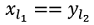Then obviously we need to find LCS for the remaining part of string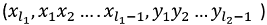and then add 1 for this character match

Else

Maximum of two case

1. LCS of the first string leaving character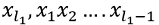and second string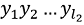2. LCS of the first string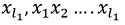and second string leaving character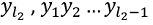Now, we need to recur down to 0. So,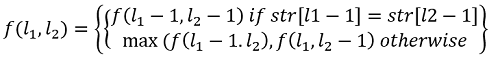Where base cases are,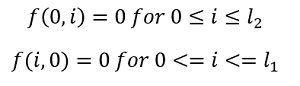If you generate this recursion tree, it will generate many overlapping sub-problems and thus, we need to reduce the re-computing. That’s why we need to convert it into dynamic programming where we will store the output of the sub-problems and we will use it to compute bigger sub-problems.

### Converting to Dynamic programing

```    1)  Initialize dp[l1+1][l2+1]  to 0
2)  Convert the base case of recursion:
for i=0 to l1
dp[i]=0;
for i=0 to l2
dp[i]=0;

3)  Fill the DP table as per recursion.
for i=1 to l1    //i be the subproblem length for str1
for j=1 to l2 //j be the subproblem length for str2
if(str1[i-1]==str2[j-1]) //xl1==yl2
dp[i][j]=dp[i-1][j-1]+1;
else
dp[i][j]=max(dp[i-1][j],dp[i][j-1]);
end for
end for
4)  The final output will be dp[l1][l2]
```

C++ Implementation:

``````#include <bits/stdc++.h>
using namespace std;

int max(int a, int b)
{
return (a > b) ? a : b;
}

int LCS(string str1, string str2)
{
int l1 = str1.length();
int l2 = str2.length();

int dp[l1 + 1][l2 + 1];

for (int i = 0; i <= l1; i++)
dp[i] = 0;
for (int i = 0; i <= l2; i++)
dp[i] = 0;

for (int i = 1; i <= l1; i++) {
for (int j = 1; j <= l2; j++) {
if (str1[i - 1] == str2[j - 1])
dp[i][j] = dp[i - 1][j - 1] + 1;
else
dp[i][j] = max(dp[i - 1][j], dp[i][j - 1]);
}
}

return dp[l1][l2];
}
int main()
{
string str1, str2;

cout << "Enter first string\n";
cin >> str1;
cout << "Enter Second string\n";
cin >> str2;

cout << "Longest Common sub-sequence length is: " << LCS(str1, str2) << endl;

return 0;
}
``````

Output

```Enter first string
includehelp
Enter Second string
letsincludeus
Longest Common sub-sequence length is: 7```